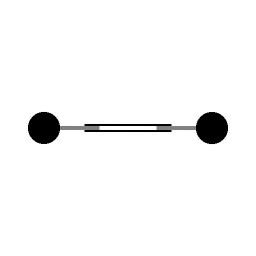# 13.4: Unequal Spacings in Pure Rotational Spectra

$$\newcommand{\vecs}{\overset { \rightharpoonup} {\mathbf{#1}} }$$ $$\newcommand{\vecd}{\overset{-\!-\!\rightharpoonup}{\vphantom{a}\smash {#1}}}$$$$\newcommand{\id}{\mathrm{id}}$$ $$\newcommand{\Span}{\mathrm{span}}$$ $$\newcommand{\kernel}{\mathrm{null}\,}$$ $$\newcommand{\range}{\mathrm{range}\,}$$ $$\newcommand{\RealPart}{\mathrm{Re}}$$ $$\newcommand{\ImaginaryPart}{\mathrm{Im}}$$ $$\newcommand{\Argument}{\mathrm{Arg}}$$ $$\newcommand{\norm}{\| #1 \|}$$ $$\newcommand{\inner}{\langle #1, #2 \rangle}$$ $$\newcommand{\Span}{\mathrm{span}}$$ $$\newcommand{\id}{\mathrm{id}}$$ $$\newcommand{\Span}{\mathrm{span}}$$ $$\newcommand{\kernel}{\mathrm{null}\,}$$ $$\newcommand{\range}{\mathrm{range}\,}$$ $$\newcommand{\RealPart}{\mathrm{Re}}$$ $$\newcommand{\ImaginaryPart}{\mathrm{Im}}$$ $$\newcommand{\Argument}{\mathrm{Arg}}$$ $$\newcommand{\norm}{\| #1 \|}$$ $$\newcommand{\inner}{\langle #1, #2 \rangle}$$ $$\newcommand{\Span}{\mathrm{span}}$$$$\newcommand{\AA}{\unicode[.8,0]{x212B}}$$

Vibrational energy which is a consequence of the oscillations/ vibrations of the nuclei along inter nuclear axis, is possible only when the distance between the nuclei is not fixed/ rigid; that means the separation between the two nuclei is flexible/ elastic (non-rigid rotator). Consequently, centrifugal force, when the molecule is rotating, tends to fly the reduced mass $$\mu$$ away from the axis of rotation. To keep the mass rotating about the axis, there must be some restoring force to counter balance the centrifugal force. The work done to supply this force is stored as potential energy. Therefore, unlike the case of rigid rotator, total energy of rotation in a molecule comprises of kinetic and the potential energy corresponding to centrifugal force of rotation.

Let $$r_e$$ is the distance between the nuclei when the separation is taken to be rigid and $$r_c$$ under the action of the centrifugal force. According to Hook’s law, restoring force is proportional to change in internuclear distance $$(r_c - r_e)\,$$, or $$= k(r_c - r_e)\,$$ which in turn, will be equal to the centrifugal force.Figure $$\PageIndex{1}$$: This animation can serve as an illustration of one of the aspects of the conservation of angular momentum. The animation is a schematic representation of two weights that are connected to each other. The two weights are circling around their common center of mass, the center of mass is stationary. The connection between the weight consists of pistons. When the connecting pistons contract, the rotation rate goes up. When the inward force exerted by the pistons relaxes the weight slide outwards, and the rotation rate goes down. (Public domain; Cleonis).

Centrifugal force,

$F_c = \mu r_c \omega^2 = L^2/\mu r_c{^3} \label{16}$

Equating the restoring force to the centrifugal force, one gets

$k(r_c - r_e) = \dfrac{L^2}{\mu r_c{^3}}$

$r_c - r_e = \dfrac{L^2}{k\mu r_c{^3}} \cong \dfrac{L^2}{k\mu r_e{^3}} \label{17}$

Total energy, on adding kinetic energy, as expressed in Equation $$\ref{17}$$ to the potential energy,

$\dfrac{1}{2}k (r_c - r_e)^2\,$

is given by

$E_r = \dfrac{L^2}{ 2\mu r_c{^2}} + \dfrac{1}{2}k\, (r_c - r_e)^2 \label{18}$

Using $$(r_c - r_e) = \Delta r\,$$ and eliminating $$r_c$$ from equation $$\ref{18}$$, one gets

\begin{align} E_r &= \dfrac{L^2}{2\mu\,\, r_e{^2}(1 + \Delta r/r_e)^2} + \dfrac{1}{2} k \Delta r^2 \\[5pt] &= \dfrac{ L^2(1 -2 \Delta r/r_e) } { 2\mu\,\, r_e{^2}} + \dfrac{1}{2} k \Delta r^2 \end{align}

using

$(1 + \Delta r/r_e)^{-2} \approx (1 -2 \Delta r/r_e)$

\begin{align} E_r &= \dfrac{L^2}{ 2\mu\,r_e{^2}} - \dfrac{L^4}{ k\mu^2\,r_e{^6}} + \dfrac{1}{2}k \dfrac{ L^4}{ k^{2\mu 2}r_e{^6}} \\[5pt] & = \dfrac{L^2}{ 2\mu\,r_e{^2}} - \dfrac{1}{2} \dfrac{ L^4}{ k\mu^2\,r_e{^6}} \label{19} \\[5pt] & = \dfrac{\hbar^2\,\,J(J + 1)}{ 2 \mu\,r_e{^2}} - \dfrac{\hbar^4\,\,J^2(J + 1)^2}{2k\mu^2\, r_e{^6}} \label{20} \end{align}

Use of Equation $$\ref{17}$$ and of the relation regarding angular momentum of a rotor

$L = \hbar\,\,\sqrt {J(J + 1)}$

has been made to obtain relation in Equation $$\ref{20}$$, which may be expressed in $$cm^{-1}$$ as

$\color{red} F(J) = \underbrace{\tilde{B} J(J + 1)}_{\text{rigid rotator term}} - \underbrace{\tilde{D} J^2(J + 1)^2 cm^﻿{-1}}_{\text{centrifugal stretching}} \label{21}$

where

$\color{red} \tilde{B} = \dfrac{ \hbar}{4\pi\,\mu\,r_e{^2}c} \,\,(\text{in cm}^{-1})$

and

$\color{red} \tilde{D} = \dfrac{\hbar^3}{4\pi k\,\mu^2\,r_e{^6}\,c} \,\,(\text{in cm}^{-1}) \label{22}$

First term in the Equation $$\ref{21}$$ is same as for the rigid rotator; second term is the consequence of the centrifugal stretching. Recall Equation $$\ref{17}$$ wherein $$k$$ is the spring constant that, as we will see in the following section, plays the same role as in the vibrational motion. In other words, centrifugal stretching constant $$\tilde{D}$$ is not only measures the influence of centrifugal force, but also hints upon the interaction between the rotational and vibrational motions.

Since $$\tilde{D}$$ is positive, it is clear from Equation $$\ref{21}$$ that the energy levels for the non-rigid rotator are slightly lower on energy scale than those of rigid rotator for the corresponding $$J$$ values; the magnitude of decrease in energy of the non-rigid rotator states increases with $$J$$ as shown in the Figure $$\PageIndex{2}$$.Figure $$\PageIndex{2}$$: Energy levels and the transitions of rigid rotator (broken red lines) & of non-rigid rotator (solid red lines). (b) resulting spectra from both the models

Consequently, on applying the selection rule $$\Delta J =\pm 1$$, rotational spectrum of a non-rigid rotator consists of a series of lines (red lines) wherein separation, unlike the case of spectral series (broken red lines) of a rigid rotator, between the consecutive rotational lines decreases with increase in $$J$$ , as shown in the Figure $$\PageIndex{2}$$. It may be noted that value of $$D$$ is very small compared to $$B$$ with the result that the influence of $$D$$ is significant only for very large $$J$$ values.

For example, for $$HCl$$ the values are $$B \sim 10.4\,\,\,cm^{-1}$$ and $$D \sim 0.0004\,cm^{-1}$$. Usually, $$D$$ is ignored in the calculations. Figure $$\PageIndex{2}$$ exaggerates the decrease in energy to visualize its effect. Nevertheless, non-rigid rotator is the model that describes the rotational motion more accurately and hence explains the spectral experimental observations not only in the microwave region but also the rotation-vibration spectra and the rotational structure of the electronic bands discussed in the later sections.